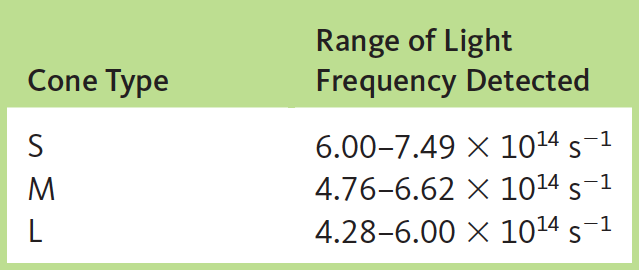# Problem: Human color vision is “produced” by the nervous system based on how three different cone receptors interact with photons of light in the eye. These three different types of cones interact with photons of different frequency light, as indicated in the following chart:What wavelength ranges (and corresponding colors) do the three types of cones detect?

###### FREE Expert Solution

Concept: Wavelength and Frequency

Wavelength and frequency can be related through the equation:

speed of light = wavelength x frequency

Since the frequency is given and the wavelength must be found, it can be rearranged:

wavelength = speed of light / frequency

Using this equation, solve for the wavelength ranges for each type of cone. Keep in mind that frequency and wavelength are inversely related, so the larger the frequency, the smaller the wavelength. Also remember that 1 m = 109 nm.

96% (394 ratings)###### Problem Details

Human color vision is “produced” by the nervous system based on how three different cone receptors interact with photons of light in the eye. These three different types of cones interact with photons of different frequency light, as indicated in the following chart:What wavelength ranges (and corresponding colors) do the three types of cones detect?